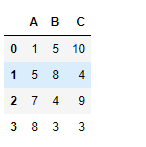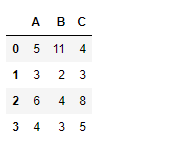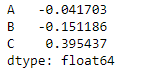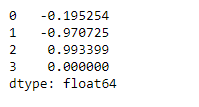Open in App
Not now

# Python | Pandas dataframe.corrwith()

• Last Updated : 16 May, 2020

Python is a great language for doing data analysis, primarily because of the fantastic ecosystem of data-centric python packages. Pandasis one of those packages and makes importing and analyzing data much easier.

Pandas` dataframe.corrwith()` is used to compute pairwise correlation between rows or columns of two DataFrame objects. If the shape of two dataframe object is not same then the corresponding correlation value will be a `NaN` value.

Syntax: DataFrame.count(axis=0, level=None, numeric_only=False)

Parameters:
other : DataFrame
axis : 0 or ‘index’ to compute column-wise, 1 or ‘columns’ for row-wise
drop : Drop missing indices from result, default returns union of all

Returns: correls : Series

Note: The correlation of a variable with itself is 1.

Example #1: Use `corrwith()` function to find the correlation among two dataframe objects along the column axis

 `# importing pandas as pd``import` `pandas as pd`` ` `# Creating the first dataframe``df1 ``=` `pd.DataFrame({``"A"``:[``1``, ``5``, ``7``, ``8``], ``                    ``"B"``:[``5``, ``8``, ``4``, ``3``],``                    ``"C"``:[``10``, ``4``, ``9``, ``3``]})`` ` `# Creating the second dataframe ``df2 ``=` `pd.DataFrame({``"A"``:[``5``, ``3``, ``6``, ``4``],``                    ``"B"``:[``11``, ``2``, ``4``, ``3``],``                    ``"C"``:[``4``, ``3``, ``8``, ``5``]})`` ` `# Print the first dataframe``print``(df1, ``"\n"``)`` ` `# Print the second dataframe``print``(df2)`Now find the correlation among the columns of the two data frames along the row axis.

 `# To find the correlation among the``# columns of df1 and df2 along the column axis``df1.corrwith(df2, axis ``=` `0``)`

Output :The output series contains the correlation between the three columns of two dataframe objects respectively.

Example #2: Use `corrwith()` function to find the correlation among two dataframe objects along the row axis

 `# importing pandas as pd``import` `pandas as pd`` ` `# Creating the first dataframe``df1 ``=` `pd.DataFrame({``"A"``:[``1``, ``5``, ``7``, ``8``],``                    ``"B"``:[``5``, ``8``, ``4``, ``3``],``                    ``"C"``:[``10``, ``4``, ``9``, ``3``]})`` ` `# Creating the second dataframe ``df2 ``=` `pd.DataFrame({``"A"``:[``5``, ``3``, ``6``, ``4``],``                    ``"B"``:[``11``, ``2``, ``4``, ``3``], ``                    ``"C"``:[``4``, ``3``, ``8``, ``5``]})`` ` `# To find the correlation among the``# columns of df1 and df2 along the row axis``df1.corrwith(df2, axis ``=` `1``)`

Output :The output series contains the correlation between the four rows of two data frame objects respectively.

My Personal Notes arrow_drop_up